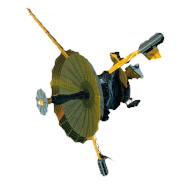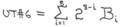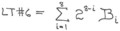GALILEO

## Galileo EPD Handbook

### Chapter 1. Instrument Summary

Galileo EPD Housekeeping Functions (continued)

Source: R. C. Moore, August 26, 1979

Table 2. Galileo EPD Subcommutated Housekeeping

Documents marked with * are not available on this website; reference APL S3 Division archives.

 Cursor Value Function Conversion Rule Comments 1 Motor housing temperature (originally analog) (See Engineering Unit Conversion Function for Temperatures in *S3D-70-000.) High and low alarm thresholds are in functions with cursor values 55 and 56. 2 Motor telemetry byte #1 Bit 1 - Scan error    0 ® no error    1 ® error (A detailed description of the motor telemetry is found in *EPD-085-79.) Scan error indicates that more pulses were delivered to the motor to make a sector change than should have been necessary. Bit 2 - Fast scan abort flag   0 ® normal   1 ® abort Fast scan abort indicates that the fast scan mode was automatically aborted due to excessive depth of discharge of motor capacitor bank. Returns to normal scan. Bit 3 - Emergency count flag   0 ® normal   1 ® emergency count number, modulo 32, occupies bits 4-8 instead of motor position data In the emergency mode, whenever the motor is between sectors, this bit goes to logic 1. Emergency count number is the number of 1.8° steps attempted since the motor was last on sector centerline. Bit 4 - Direction indicator   0 ® counterclockwise   1 ® clockwise Clockwise means sector numbers are increasing. Bit 5 - Centerline indicator   0 ® off center   1 ® on center Marks center (±1.3°) of sector. Bits 6-8 - Motor position code   P = 4B6 + 2B7 + B8 P is the sector number. 3 Motor telemetry byte #2 Bit 1 - Emergency mode indicator   0 ® normal   1 ® emergency In emergency mode, motor moves one step per trigger instead of one sector per trigger. Bit 2 - Open loop mode indicator   0 ® closed loop   1 ® open loop In closed loop mode, the motor moves until the next centerline is found or an automatic cease scan occurs. In open loop mode, a fixed number of steps is applied to the motor, to change sectors. Bit 3   Modified scan (closed loop mode)   0 ® normal scan using end sectors 0 and 7   1 ® Modified scan using end sectors programmed by ground command Limited scan (open loop mode)   0 ® normal scan   1 ® limited scan For detailed descriptions of normal scan and limited scan, see *EPD-085-79. Bit 4 - Fast scan   0 ® normal scan   1 ® fast scan Fast scan: motor receives triggers every two logical records (every 2-2/3 seconds). Bit 5  Go to sector N (closed loop mode)   0 ® normal   1 ® go to sector N and stay there Respond to N triggers (open loop mode)   0 ® normal   1 ® stop scanning after N triggers Value of N is found in bits 6, 7, and 8. Bits 6-8 - "N" N = 4B6 + 2B7 + B8 4 Motor telemetry byte #3 Bit 1 - Alternate step rate mode   0 ® normal   1 ® alternate step rate Normal: ~50 steps/sec (~90°/sec) Alternate: ~60 steps/sec (108°/sec) Bits 2-4 - CCW end-sector   Sccw = 4B2 + 2B3 + B4 Bits 2-4 are not used in open loop mode. Bit 5 - Chicken Mode (closed loop mode)   0 ® normal   1 ® chicken mode "Chicken Mode" is a mode in which most of the time is spent behind the shield (sector 0). (Not used in open loop mode.) Bits 6-8 - CW end-sector   Scw = 4B6 + 2B7 + B8 Bits 6-8 are not used in open loop mode. 5 Autocalibrator index #1 I1 = 64B2 + 32B3 + 16B4 + 8B5 + 4B6 + 2B7 + B8 B1 is ignored. This index identifies contents of next subcommutated function, according toTable 1 and *EPD-050-79. 6 Autocalibrator AGC voltage #1 (originally analog) AGC1 = 2.56B1 + 1.28B2 + .64B3 + .32B4 + .16B5 + .08B6 + .04B7 + .02B8 0 volts £ AGC £ 5.1 volts 7 Motor telemetry byte #4 N = 128B1 + 64B2 + 32B3 + 16B4 + 8B5 + 4B6 + 2B7 + B8 N = number of invalid motor commands, modulo 256. 8 Motor telemetry byte #5 Bits 1 & 2 not used. Bits 3-7 - step count (closed loop mode)   SC = 16B3 + 8B4 + 4B5 + 2B6 + B7 Bits 3-7 not used in open loop mode. Step count is the number of steps required to make the most recent sector change. Bit 8 - cease scan flag (closed loop mode)   0 ® normal   1 ® cease scan Bit 8 not used in open loop mode. "Cease scan" indicates that the motor was unable to find a centerline during a closed loop sector change attempt. Motor automatically tries to return to centerline of previous sector. 9 LEMMS telescope temperature (originally analog) (See Engineering Unit Conversion Function for Temperatures in *S3D-79-000.) High and low alarm thresholds are in functions with cursor values 61 and 62. 10 Number of invalid bus commands, modulo 256 NIC = 128B1 + 64B2 + 32B3 + 16B4 + 8B5 + 4B6 + 2B7 + B8 NIC is the sum of invalid data system commands and invalid scanning system commands. Function at cursor 7 is scanning system invalid command count. 11 Autocalibrator index #2 (Same as for cursor #5) See *EPD-050-79. 12 Autocalibrator AGC voltage #2 (originally analog) (Same as for AGC1 - see cursor #6.) 13 Memory dump cursor (8 MSBs) MDC = 32768B1 + 16384B2 + 8192B3 + 4096B4 + 2048B5 + 1024B6 + 512B7 + 256B8 + (least significant half) Least significant half of MDC is in channels 9 and 95 of the EPD logical record (see *S3D-79-093). 14 Motor dwell period MDP = 170.67B1 + 85.33B2 + 42.67B3 + 21.33B4 + 10.67B5 + 5.33B6 + 2.67B7 + 1.33B8 MDP is programmable by ground command in integer multiples of 1.333 seconds. During accelerated scan, MDP = 2.667. Default (initial) value for MDP is 20 seconds. 15 CMS telescope temperature (originally analog) (See Engineering Unit Conversion Function for Temperatures in *S3D-79-000.) High and low alarm thresholds are in functions with cursor values 67 and 68. 16 Supervisory data parity error count SDP = 128B1 + 64B2 + 32B3 + 16B4 + 8B5 + 4B6 + 2B7 + B8 SDP is the number of parity errors detected by EPD bus adapter since last power-on, modulo 256. 17 Autocalibrator index #3 (Same as for cursor #5) See *EPD-050-79. 18 Autocalibrator AGC voltage #3 (originally analog) (Same as for AGC1 - see cursor #6.) 19 Upper memory checksum limit (8 MSBs) UMCL = 32768B1 + 16384B2 + 8192B3+ 4096B4 + 2048B5 + 1024B6 + 512B7 + 256B8 + (least significant half) Least significant half of UMCL is in function with cursor value = 20. 20 Upper memory checksum limit (8 LSBs) UMCL = 128B1 + 64B2 + 32B3 + 16B4+ 8B5 + 4B6 + 2B7 + B8 + (most significant half) Most significant half of UMCL is in function with cursor value = 20. 21 Main electronics temperature (originally analog) (See Engineering Unit Conversion Function for Temperatures in *S3D-79-000.) High and low alarm thresholds are in functions with cursor values 73 and 74. 22 Memory checksum MCM = 128B1 + 64B2 + 32B3 + 16B4 + 8B5 + 4B6 + 2B7 + B8 23 Autocalibrator index #4 (Same as for cursor #5) See *EPD - 050-79. 24 Autocalibrator AGC voltage #4 (originally analog) (Same as for AGC1 - see cursor #6.) 25 Lower memory checksum limit (8 MSBs) LMCL = 32768B1 + 16384B2 + 8192B3+ 4096B4 + 2048B5 + 1024B6 + 512B7 + 256B8 + (least significant half) Least significant half of LMCL is in function with cursor value = 26. 26 Lower memory checksum limit (8 LSBs) LMCL = 128B1 + 64B2 + 32B3 + 16B4 + 8B5 + 4B6 + 2B7 + B8 + (most significant half) Most significant half of LMCL is in function with cursor value = 25. 27 EPD input current (originally analog) (See Engineering Unit Conversion Function for Current in *S3D-79-000.) High and low alarm thresholds are in functions with cursor values 49 and 50. 28 EPD data parity error count EDP = 128B1 + 64B2 + 32B3 + 16B4 + 8B5 + 4B6 + 2B7 + B8 EDP is the number of parity errors detected by EPD bus adapter during EPD bus transactions since last power-on, modulo 256. 29 Autocalibrator index #5 (Same as for cursor #5) See *EPD-050-79. 30 Autocalibrator AGC voltage #5 (originally analog) (Same as for AGC1 - see cursor #6.) 31 LEMMS PHA A K1 AK1 = 128B1 + 64B2 + 32B3 + 16B4 + 8B5 + 4B6 + 2B7 + B8 0 £ A K1 £ 192 32 LEMMS PHA A K2 AK2 = 128B1 + 64B2 + 32B3 + 16B4 + 8B5 + 4B6 + 2B7 + B8 0 £ A K2 £ 2 33 +60 volts bias (originally analog) (See Engineering Unit Conversion Function in *S3D-79-000.) 34 Power switch status byte #1 Bit n = 0 ® LEMMS AMP (9-n) off Bit n = 1 ® LEMMS AMP (9-n) on 1  £ n  £ 8 (See *S3D-79-104.) 35 Autocalibrator index #6 (Same as for cursor #5) See *EPD-050-79. 36 Autocalibrator AGC voltage #6 (originally analog) (Same as for AGC1 - see cursor #6.) 37 LEMMS PHA E K1 EK1 = 128B1 + 64B2 + 32B3 + 16B4 + 8B5 + 4B6 + 2B7 + B8 0 £ E K1 £ 192 38 LEMMS PHA E K2 EK2 = 128B1 + 64B2 + 32B3 + 16B4 + 8B5 + 4B6 + 2B7 + B8 0 £ E K2 £ 2 39 +20 volts bias (originally analog) (See Engineering Unit Conversion Function in *S3D-79-000.) 40 Power switch status byte #2 B1 - spare B2 = 1 ® calibrator on B2 = 0 ® calibrator off B3 = 1 ® PHA on B3 = 0 ® PHA off B4 = 0 ® TOF on B4 = 0 ® TOF off B5 = 1 ® CMS electronics on B5 = 0 ® CMS electronics offCMS electronics off B6 = 1 ® Detector bias high B6 = 0 ® Detector bias normalDetector bias normal B7 = 1 ® CMS J' bias on (+20V) B7 = 0 ® CMS J' bias offCMS J' bias off B8 = 1 ® LEMMS A detector bias on (+60V) B8 = 0 ® LEMMS A detector bias off (See *S3D-79-104.) 41 Autocalibrator index #7 (Same as for cursor #5) See *EPD-050-79. 42 Autocalibrator AGC voltage #7 (originally analog) (Same as for AGC1 - see cursor #6.) 43 LEMMS PHA F K1 FK1 = 128B1 + 64B2 + 32B3 + 16B4 + 8B5 + 4B6 + 2B7 + B8 0 £ F K1 £ 192 44 LEMMS PHA F K2 FK2 = 128B1 + 64B2 + 32B3 + 16B4 + 8B5 + 4B6 + 2B7 + B8 0 £ F K2 £ 2 45 -15 Volts power (originally analog) (See Engineering Unit Conversion Function in *S3D-79-000.) 46 Power switch status byte #3 B1 = 1 ® LEMMS -10V power on B1 = 0 ® LEMMS -10V power off B2 = 1 ® motor on B2 = 0 ® motor off B3 = 1 ® motor RAM exchanged  B3 = 0 ® motor RAM normal B4 B5 B6 - spares B7 = 1 ® LEMMS E11LEMMS E11 thresh. high B7 = 0 ® LEMMS E11 thresh. normal B8 = 1 ® LEMMS A1 thresh. high B8 = 0 ® LEMMS A1 thresh. normal 47 Autocalibrator index #8 (Same as for cursor #5) See *EPD-050-79. 48 Autocalibrator AGC voltage #8 (originally analog) (Same as for AGC1 - see cursor #6.) 49 Upper alarm threshold for EPD input current UTIC = 128B1 + 64B2 + 32B3 + 16B4 + 8B5 + 4B6 + 2B7 + B8 Engineering unit conversion is in *S3D-79-000. 50 Lower alarm threshold for EPD input current LTIC = 128B1 + 64B2 + 32B3 + 16B4 + 8B5 + 4B6 + 2B7 + B8 See comment at cursor 49. 51 +10 Volts power (originally analog) (See Engineering Unit Conversion Function in *S3D-79-000.) 52 Power switch status byte #4 B1 = 1 ® TOF logical condition off B1 = 0 ® TOF logical condition on B2 - spare B3 = 1 ® CMS analog J'K' on B3 = 0 ® CMS analog J'K' off B4 = 1 ® CMS analog L on B4 = 0 ® CMS analog L off B5 = 1 ® CMS analog Jc on B5 = 0 ® CMS analog Jc off B6 = 1 ® CMS analog Jb on B6 = 0 ® CMS analog Jb off B7 = 1 ® CMS analog Ja on B7 = 0 ® CMS analog Ja off B8 = 1 ® CMS prime select on B8 = 0 ® CMS prime select off 53 Autocalibrator index #9 (Same as for cursor #5) See *EPD-050-79. 54 Autocalibrator AGC voltage #9 (originally analog) (Same as for AGC1 - see cursor #6.) 55 Upper alarm threshold for motor temperature UTMT = 128B1 + 64B2 + 32B3 + 16B4+ 8B5 + 4B6 + 2B7 + B8 See comment at cursor #49. 56 Lower alarm threshold for motor temperature LTMT = 128B1 + 64B2 + 32B3 + 16B4 + 8B5 + 4B6 + 2B7 + B8 See comment at cursor #49. 57 +6 Volts power (originally analog) (See Engineering Unit Conversion Function in *S3D-79-000.) 58 PHA control byte B1 = EP1        priority = 2 EP1 + EP0 B2 = EP0          "                 "        " B3 = 0 ® priority override B3 = 1 ® priority normal B4 = 1 ® PHA reset B4 = 0 ® PHA normal  B5 = LS1      LEMMS select = 2 LS1 + LS0 B6 = LS0         "                       "        " B7 = 0 ® P read active B7 = 1 ® P read inactive B8 = 0 ® LEMMS mode B8 = 1 ® CMS mode 59 Autocalibrator index #10 (Same as for cursor #5) See *EPD-050-79. 60 Autocalibrator AGC voltage #10 (originally analog) (Same as for AGC1 - see cursor #6.) 61 Upper alarm threshold for LEMMS telescope temperature UTLT = 128B1 + 64B2 + 32B3 + 16B4 + 8B5 + 4B6 + 2B7 + B8 See comment at cursor #49. 62 Lower alarm threshold for LEMMS telescope temperature LTLT = 128B1 + 64B2 + 32B3 + 16B4 + 8B5 + 4B6 + 2B7 + B8 See comment at cursor #49. 63 +3 Volts power (originally analog) (See Engineering Unit Conversion Function in *S3D-79-000.) 64 (spare) 65 Autocalibrator index #11 (Same as for cursor #5) See *EPD-050-79. 66 Autocalibrator AGC voltage #11 (originally analog) (Same as for AGC1 - see cursor #6.) 67 Upper alarm threshold for CMS telescope temperature UTCT = 128B1 + 64B2 + 32B3 + 16B4 + 8B5 + 4B6 + 2B7 + B8 See comment at cursor #49. 68 Lower alarm threshold for CMS telescope temperature LTCT = 128B1 + 64B2 + 32B3 + 16B4 + 8B5 + 4B6 + 2B7 + B8 See comment at cursor #49. 69 -3 Volts power (originally analog) (See Engineering Unit Conversion Function in *S3D-79-000.) 70 (spare) 71 Autocalibrator index #12 (Same as for cursor #5) See *EPD-050-79. 72 Autocalibrator AGC voltage #12 (originally analog) (Same as for AGC1 - see cursor #6.) 73 Upper alarm threshold for main electronics temperature UTET = 128B1 + 64B2 + 32B3 + 16B4 + 8B5 + 4B6 + 2B7 + B8 See comment at cursor #49. 74 Lower alarm threshold for main electronics temperature LTET = 128B1 + 64B2 + 32B3 + 16B4 + 8B5 + 4B6 + 2B7 + B8 See comment at cursor #49. 75 -6 Volts power (originally analog) (See Engineering Unit Conversion Function in S*3D-79-000.) 76 (spare) 77 Autocalibrator index #13 (Same as for cursor #5) See *EPD-050-79. 78 Autocalibrator AGC voltage #13 (originally analog) (Same as for AGC1 - see cursor #6.) 79 Upper alarm threshold #680 Lower alarm threshold #681 -10 Volts power (originally analog) (See Engineering Unit Conversion Function in *S3D-79-000.) 82 (spare) 83 Autocalibrator index #14 (Same as for cursor #5) See *EPD-050-79. 84 Autocalibrator AGC voltage #13 (originally analog) (Same as for AGC1 - see cursor #6.) 85 (spare) 86 (spare) 87 Dust cover deployment (originally analog) DC = 128B1 + 64B2 + 32B3 + 16B4 + 8B5 + 4B6 + 2B7 + B8 0 £ DC £ 32 ® covers closed.   33 £ DC £ 96 ® sector 1 cover open, sector 7 cover closed.   97 £ DC £ 137 ® sector 1 cover closed, sector 7 cover open.   138 £ DC £ 255 ® both covers open. 88 (spare) 89 (spare) 90 (spare) 91 (spare)